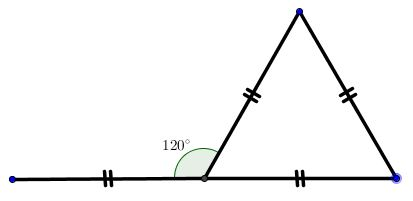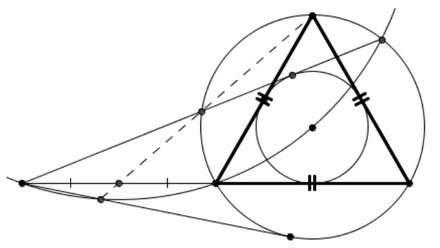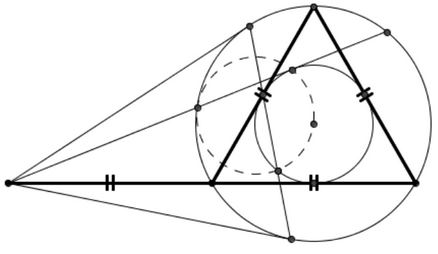# Seven Problems in Equilateral Triangle

The following has been communicated to me in private correspondence by Emmanuel Antonio José García (15 February, 2014).

The basic configuration consists of an equilateral triangle with the base extended to twice its length:The statements appear as dashed lines; the lines that look tangent to a circle are indeed tangent. Solutions are welcome.

### Problem 1Solution

### Problem 2Solution

### Problem 3Solution

### Problem 4Solution

### Problem 5Solution

### Problem 6Solution

### Problem 7Solution• Angle Trisectors on Circumcircle
• Equilateral Triangles On Sides of a Parallelogram
• Pompeiu's Theorem
• Pairs of Areas in Equilateral Triangle
• The Eutrigon Theorem
• Equilateral Triangle in Equilateral Triangle
• Spiral Similarity Leads to Equilateral Triangle
• Parallelogram and Four Equilateral Triangles
• A Pedal Property in Equilateral Triangle
• Miguel Ochoa's van Schooten Like Theorem
• Two Conditions for a Triangle to Be Equilateral
• Incircle in Equilateral Triangle
• When Is Triangle Equilateral: Marian Dinca's Criterion
• Barycenter of Cevian Triangle
• Excircle in Equilateral Triangle
• Converse Construction in Pompeiu's Theorem
• Wonderful Trigonometry In Equilateral Triangle
• 60o Angle And Importance of Being The Other End of a Diameter
• One More Property of Equilateral Triangles
• Van Khea's Quickie
• Equilateral Triangle from Three Centroids
•